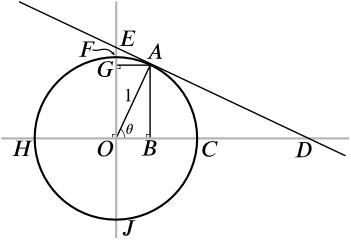#### You may also likeThis comes in two parts, with the first being less fiendish than the second. Itâ€™s great for practising both quadratics and laws of indices, and you can get a lot from making sure that you find all the solutions. For a real challenge (requiring a bit more knowledge), you could consider finding the complex solutions.### Discriminating

You're invited to decide whether statements about the number of solutions of a quadratic equation are always, sometimes or never true.This will encourage you to think about whether all quadratics can be factorised and to develop a better understanding of the effect that changing the coefficients has on the factorised form.

# Have a Sine

##### Age 16 to 18Challenge LevelThis resource is from Underground Mathematics.

The trigonometric functions with which we are most familiar are sine and cosine. From them, we can obtain tangent, and also the reciprocals secant, cosecant and cotangent.

In the past, mathematicians have used many other trigonometric functions too. Some of these are illustrated below.Find the following lengths, in terms of $\sin\theta$ and $\cos \theta$.

• $OB$
• $AB$
• $BC$ (this was known as the versed sine or versine of $\theta$, written $\mathop{\mathrm{versin}}\theta$)
• $OD$
•  $CD$ (this was known as the exsecant of $\theta$, written $\mathop{\mathrm{exsec}}\theta$)
• $AD$
• $AE$
• $AG$
• $GO$
• $FG$ (this was known as the coversed sine or coversine of $\theta$, written $\mathop{\mathrm{coversin}}\theta$ or $\mathop{\mathrm{covers}}\theta$ or $\mathop{\mathrm{cvs}}\theta$)
• $EF$ (this was known as the excosecant of $\theta$, written $\mathop{\mathrm{excosec}}\theta$ or $\mathop{\mathrm{excsc}}\theta$)

Can you find line segments on the diagram that have the following lengths?

• The versed cosine or vercosine of $\theta$, written as $\mathop{\mathrm{vercos}}\theta$, which is defined by $$\mathop{\mathrm{vercos}}\theta = 1 + \cos\theta$$
• The coversed cosine or covercosine of $\theta$, written as $\mathop{\mathrm{covercos}}\theta$, which is defined by $$\mathop{\mathrm{covercos}}\theta = 1 + \sin\theta$$

We know that $\cos\theta = \sin\left(\frac{\pi}{2} - \theta\right)$.

• How are versine and coversine related?
• How are vercosine and covercosine related?
• What do you think that the prefix "co-" might indicate here?

This is an Underground Mathematics resource.

Underground Mathematics is hosted by Cambridge Mathematics. The project was originally funded by a grant from the UK Department for Education to provide free web-based resources that support the teaching and learning of post-16 mathematics.

Visit the site at undergroundmathematics.org to find more resources, which also offer suggestions, solutions and teacher notes to help with their use in the classroom.